# RD Sharma Solutions for Class 8 Maths Chapter 3 - Squares and Square Roots Exercise 3.3

RD Sharma Solutions for Class 8 Maths Exercise 3.3 Chapter 3, Squares and Square Roots are provided here. The questions present in this exercise have been solved by BYJU’S subject experts, and this will help students in solving the questions without any obstacles. The RD Sharma Solutions Class 8 are prepared according to the concepts covered in the textbook to understand each and every problems thoroughly. Exercise 3.3 of RD Sharma Solutions for Class 8 helps students to understand the concepts of some shortcuts to find squares which include column method, visual method and the diagonal method for squaring a two-digit number.

## Download the pdf of RD Sharma Solutions For Class 8 Maths Chapter 3 Squares and Square Roots Exercise 3.3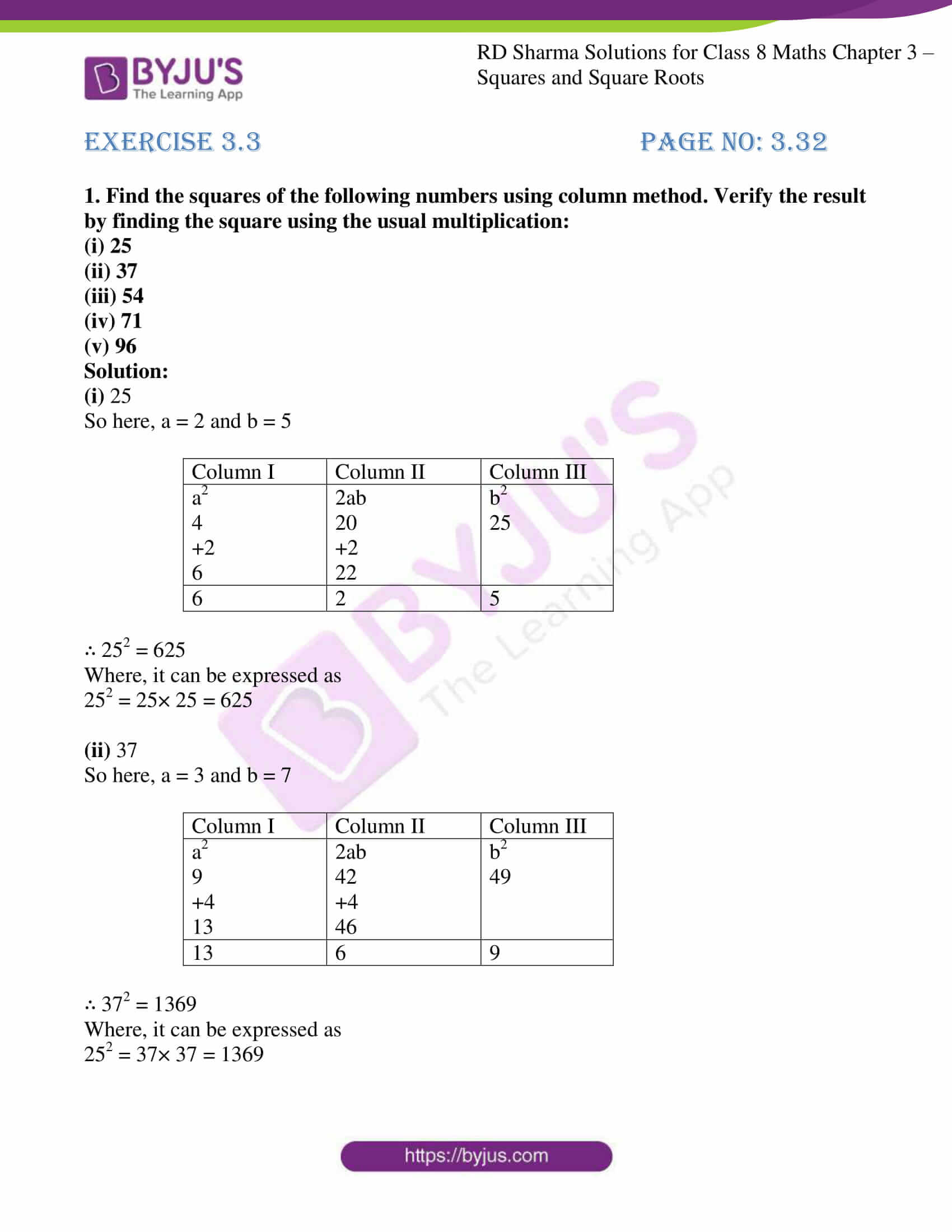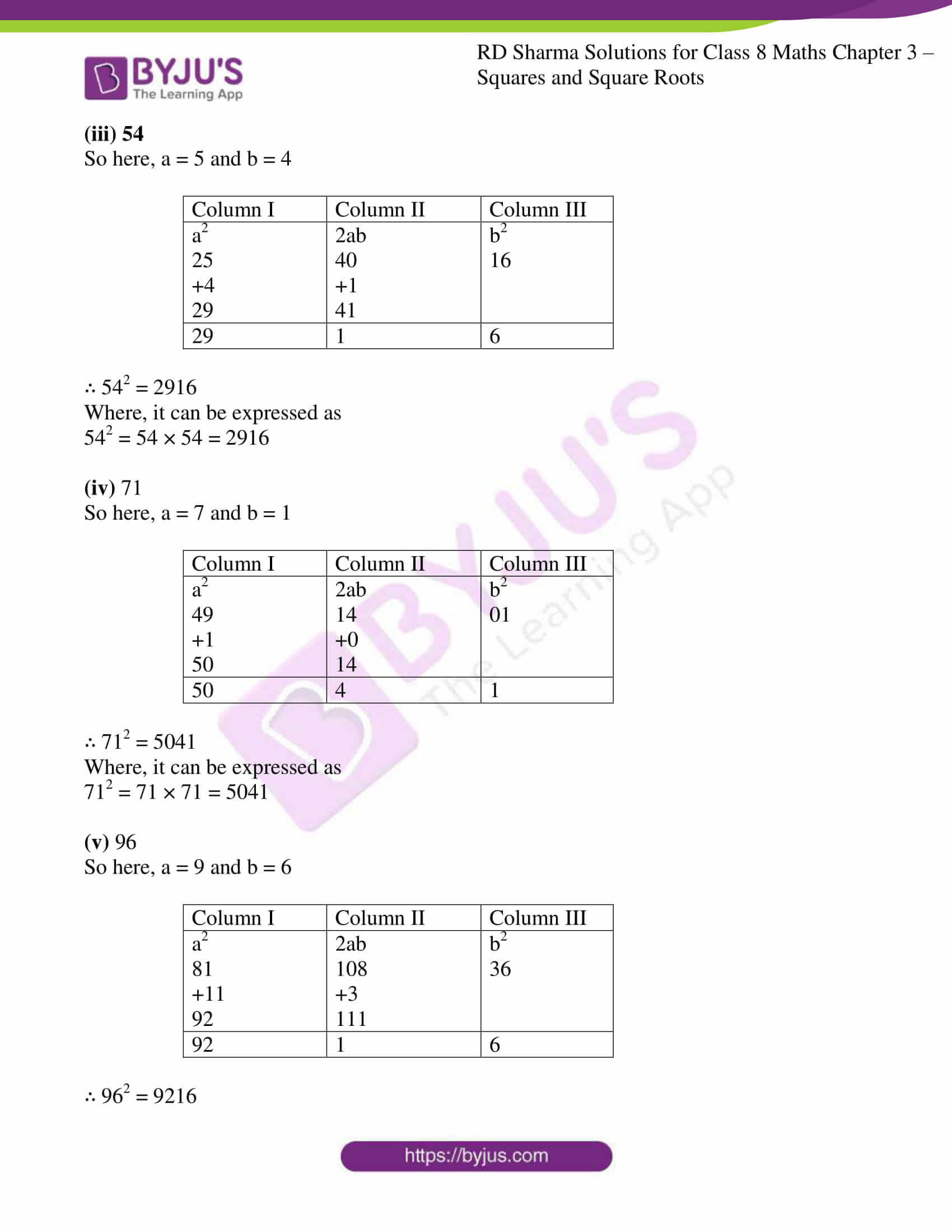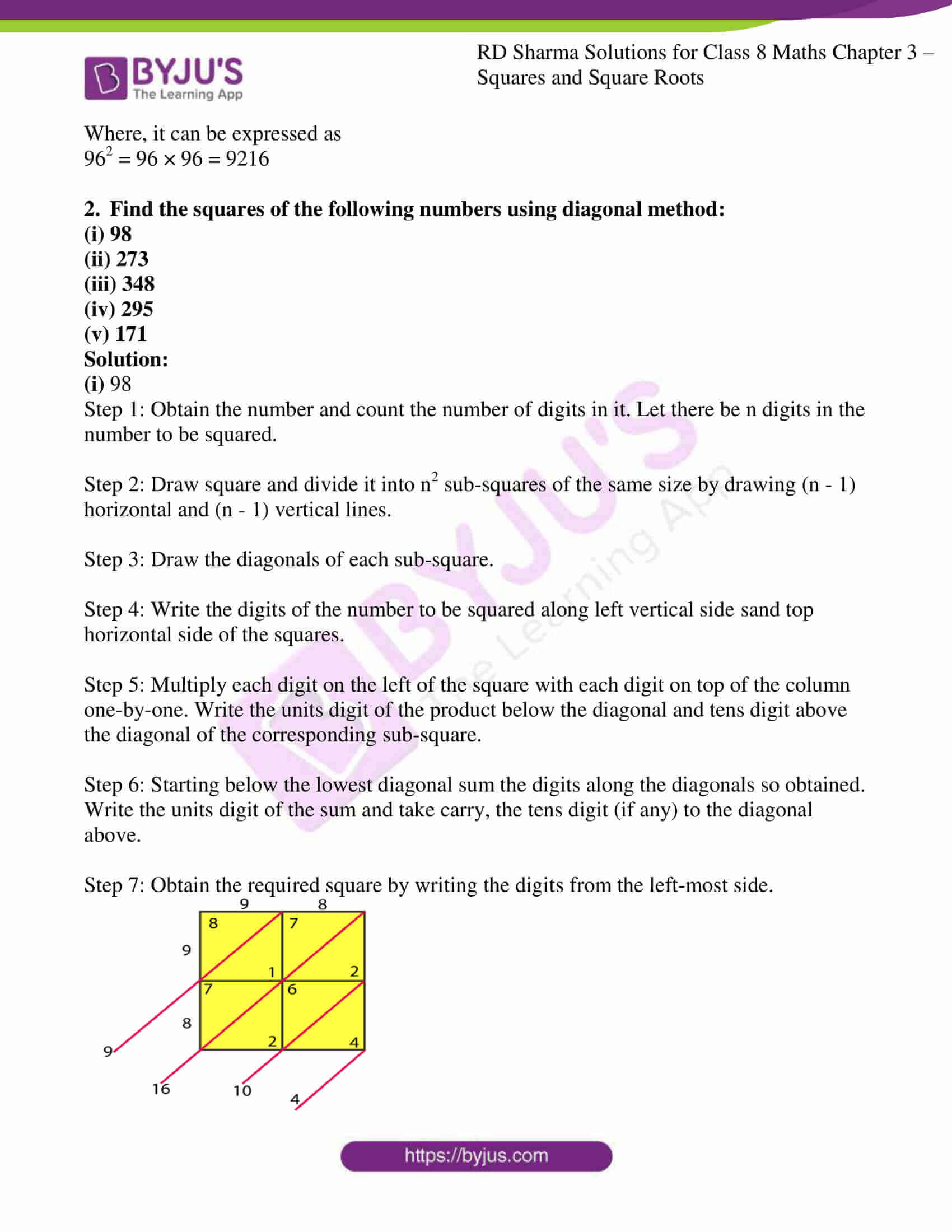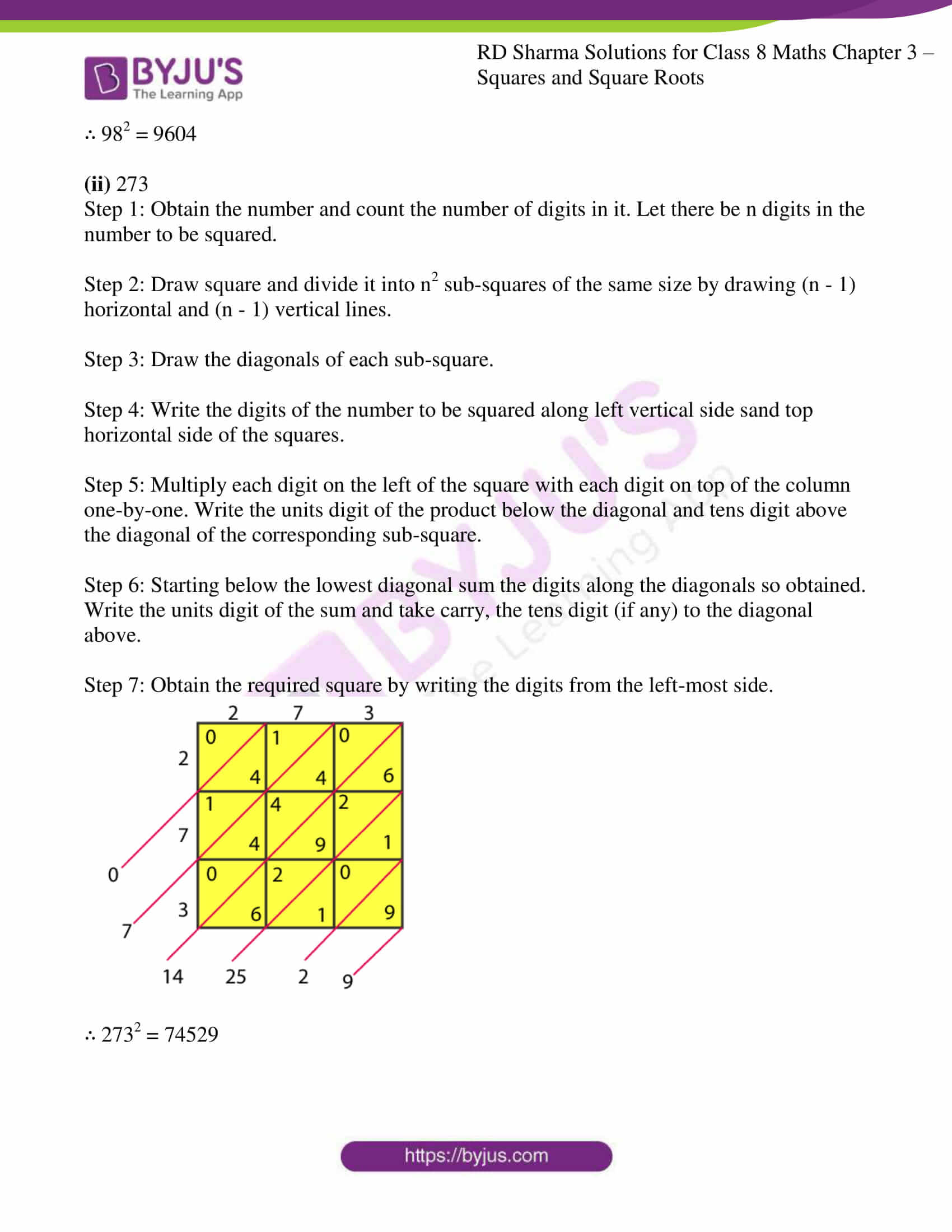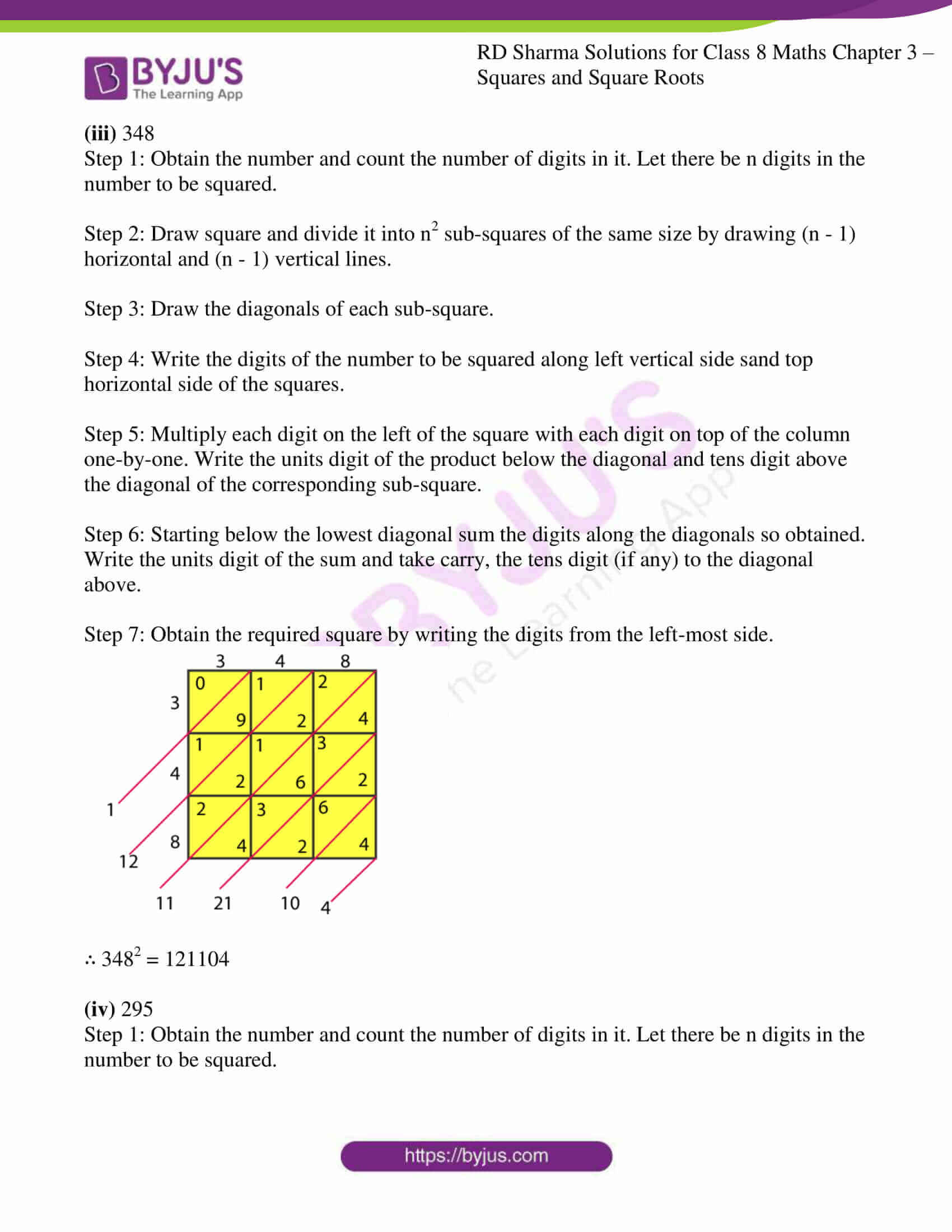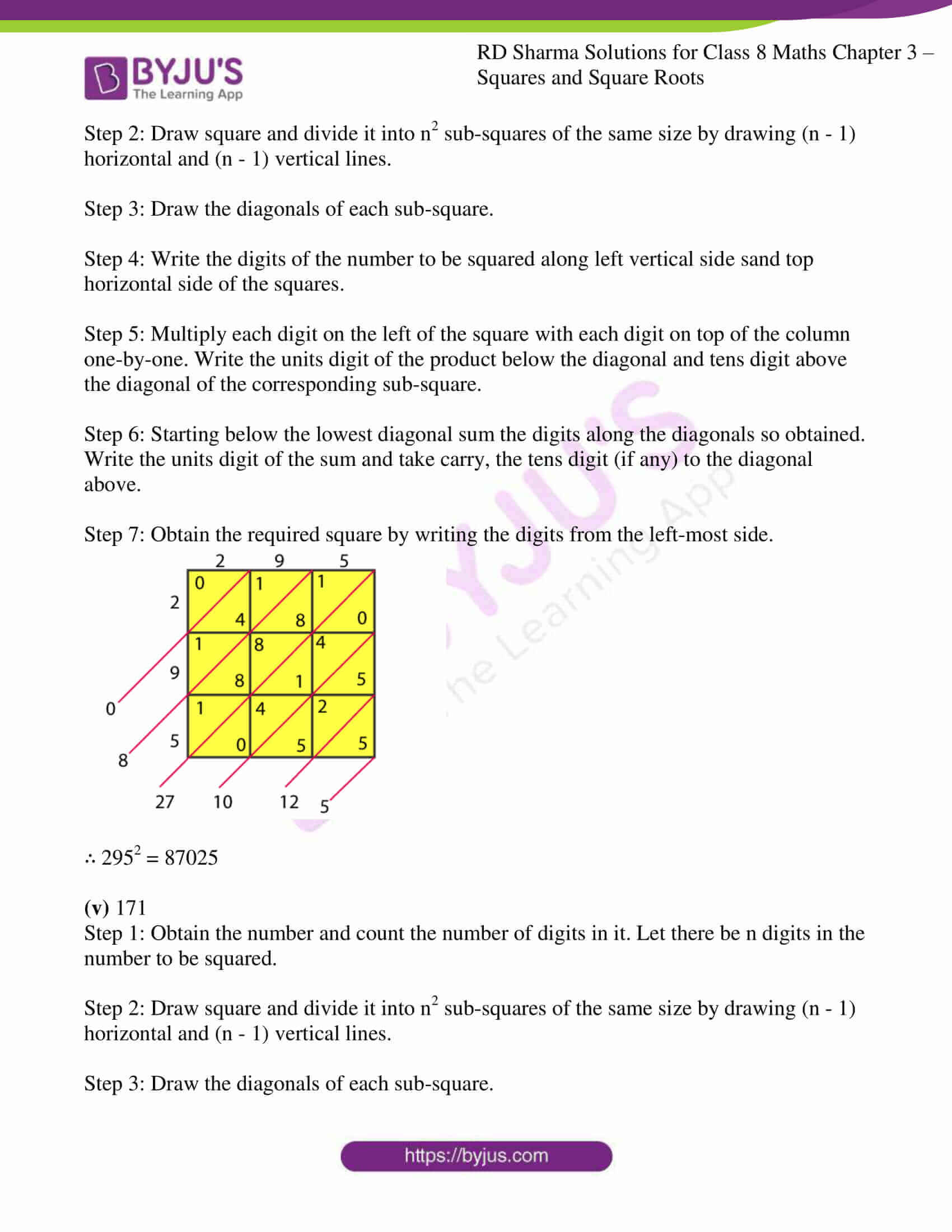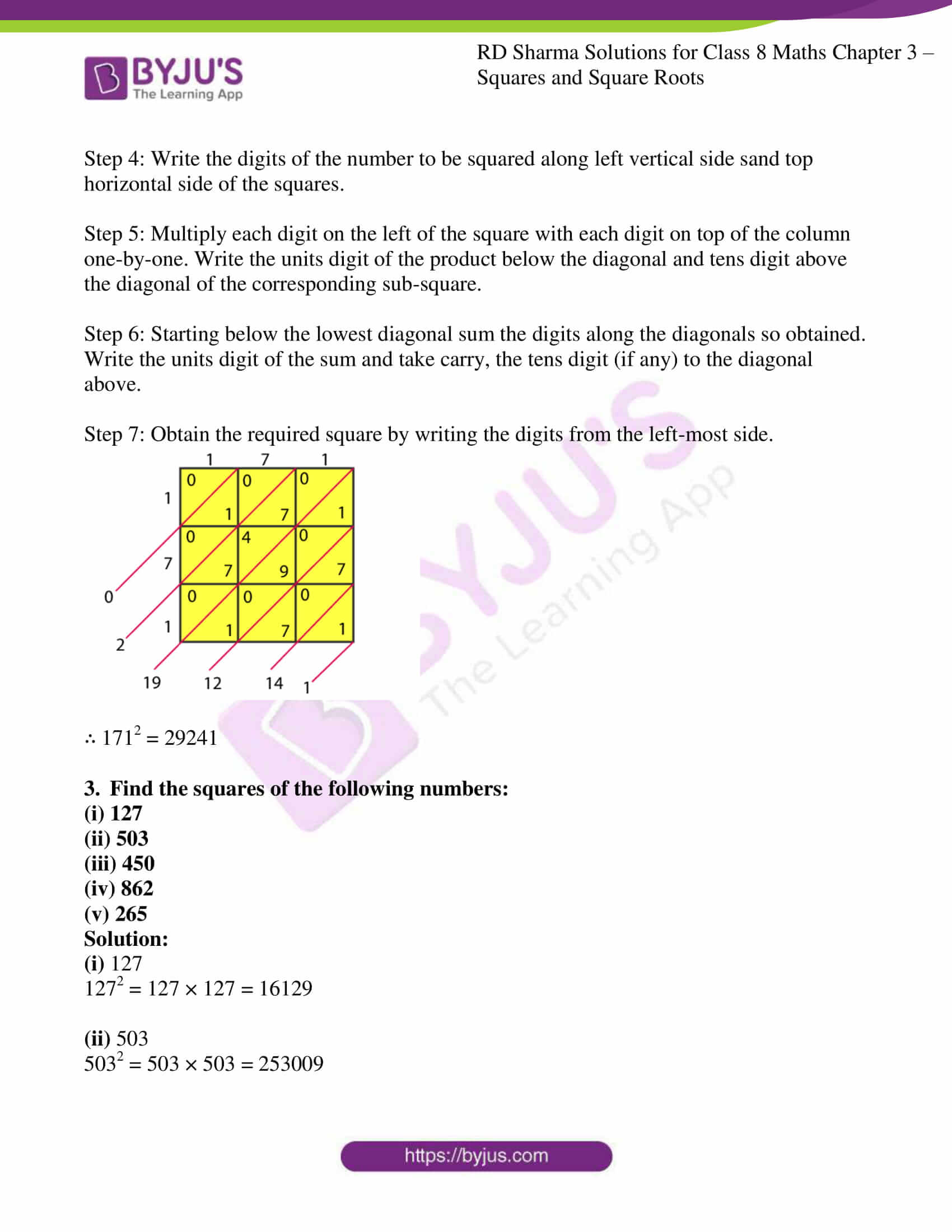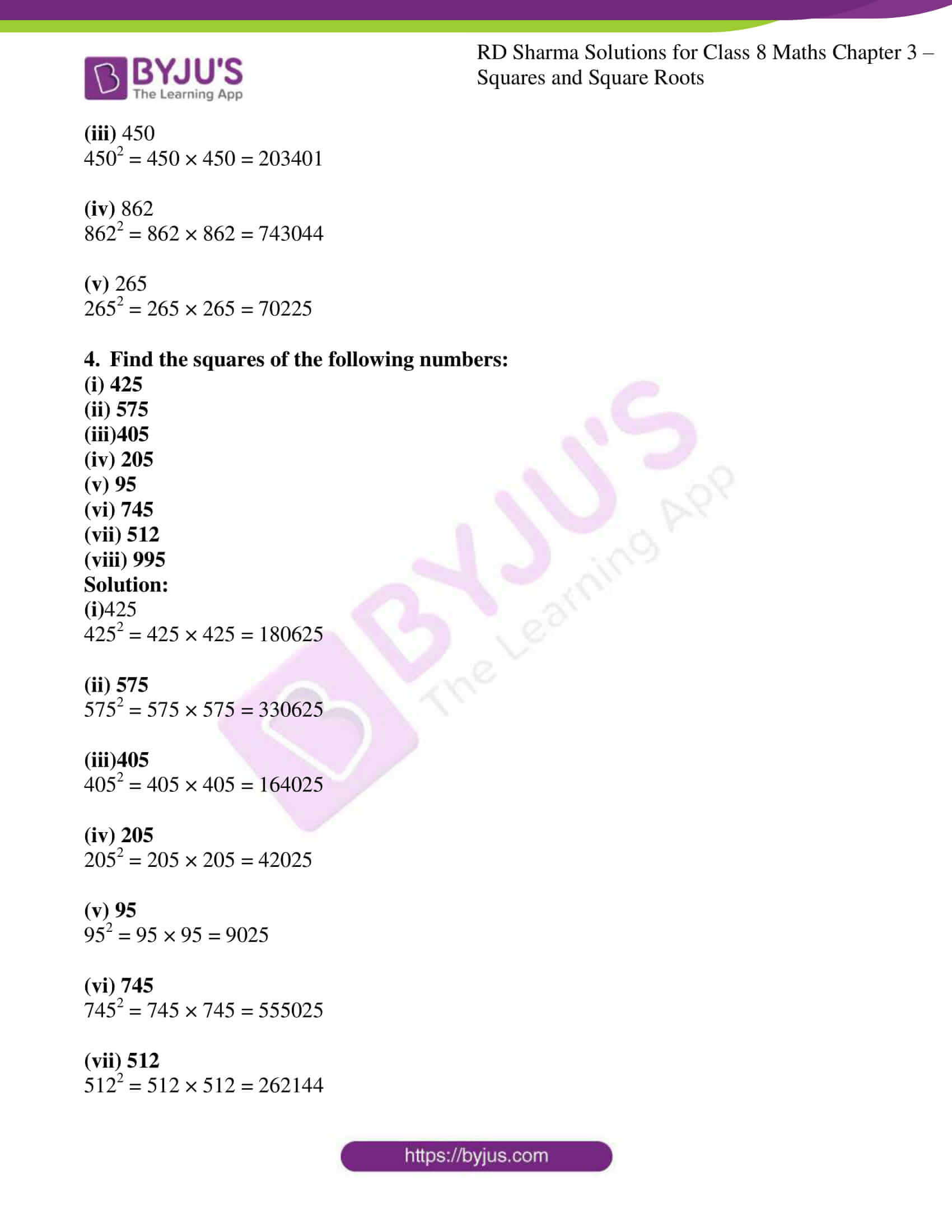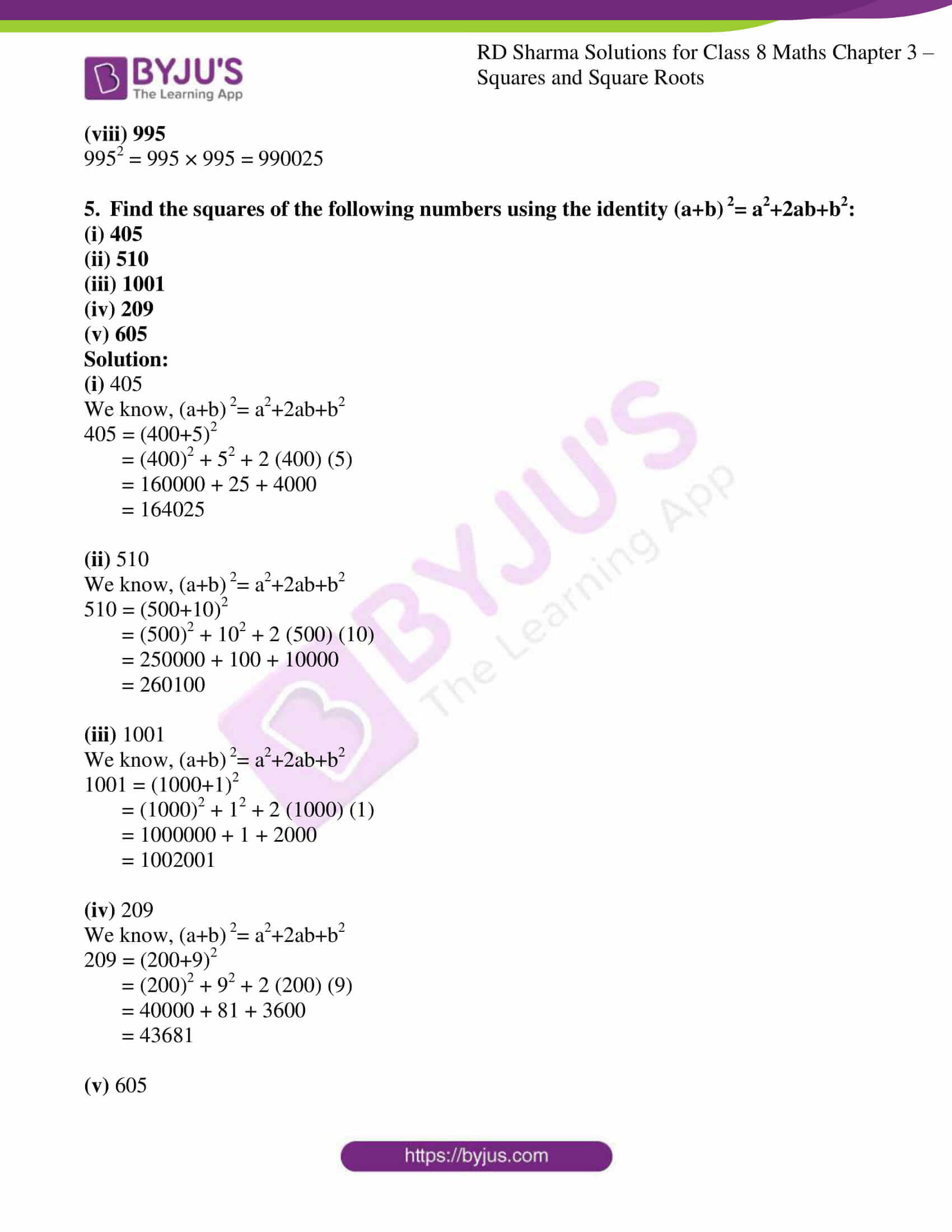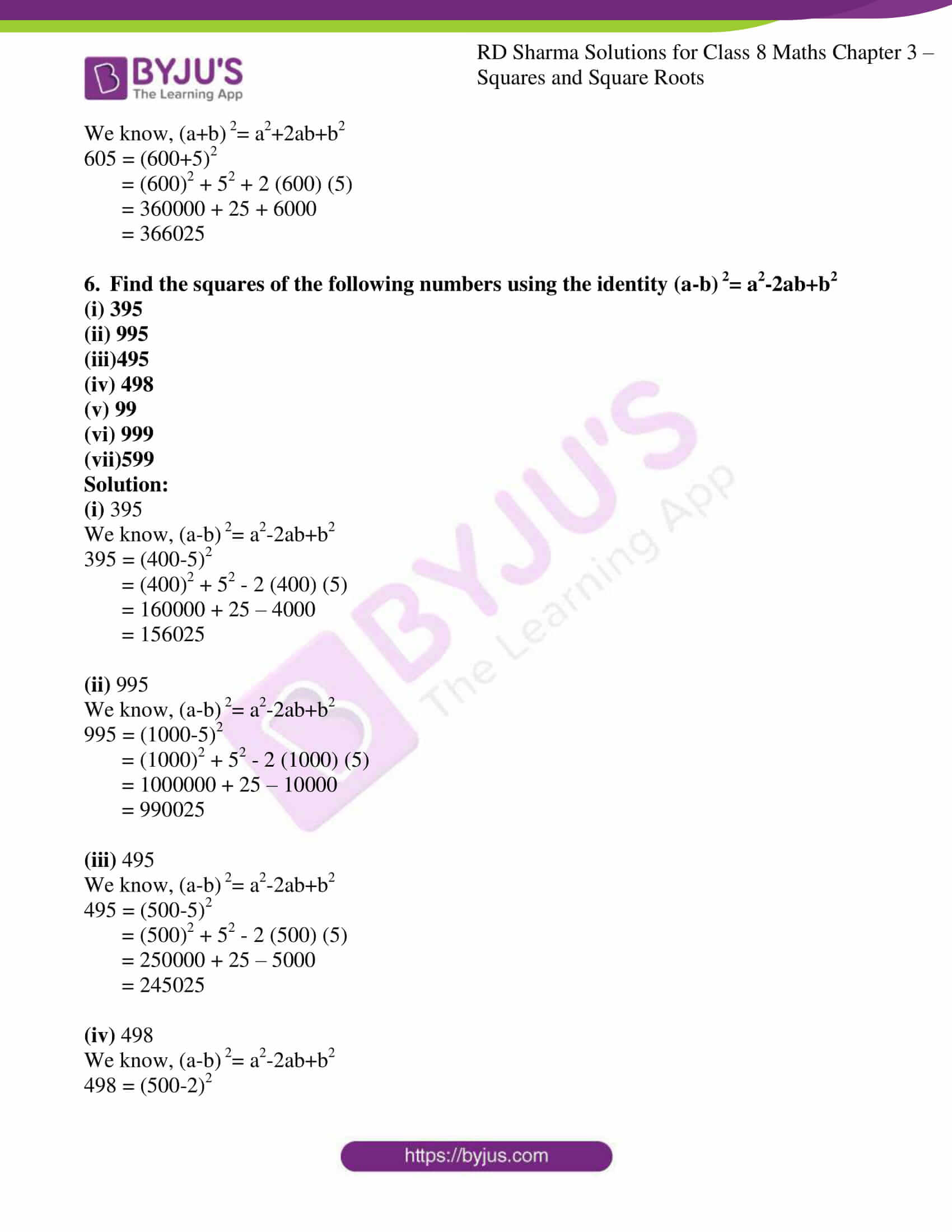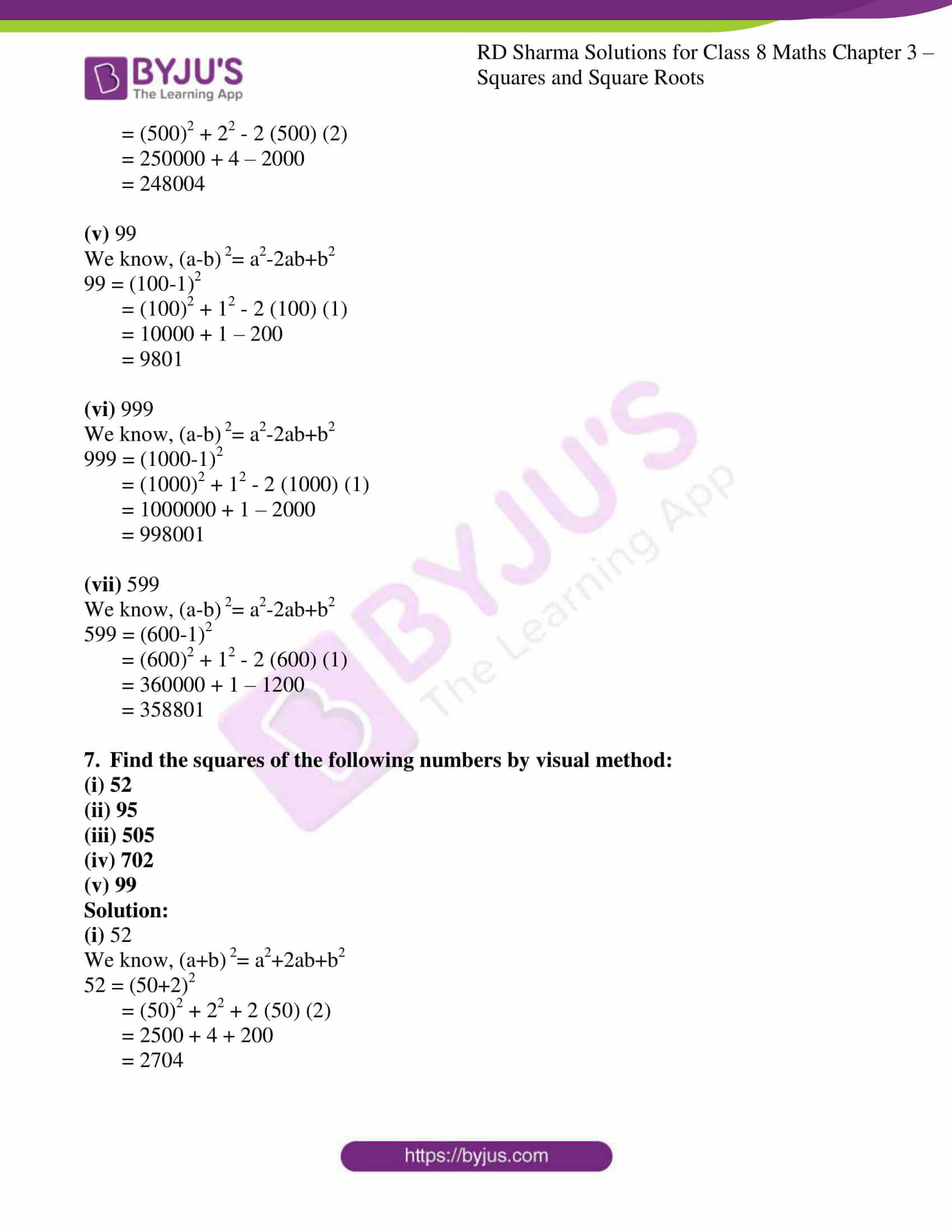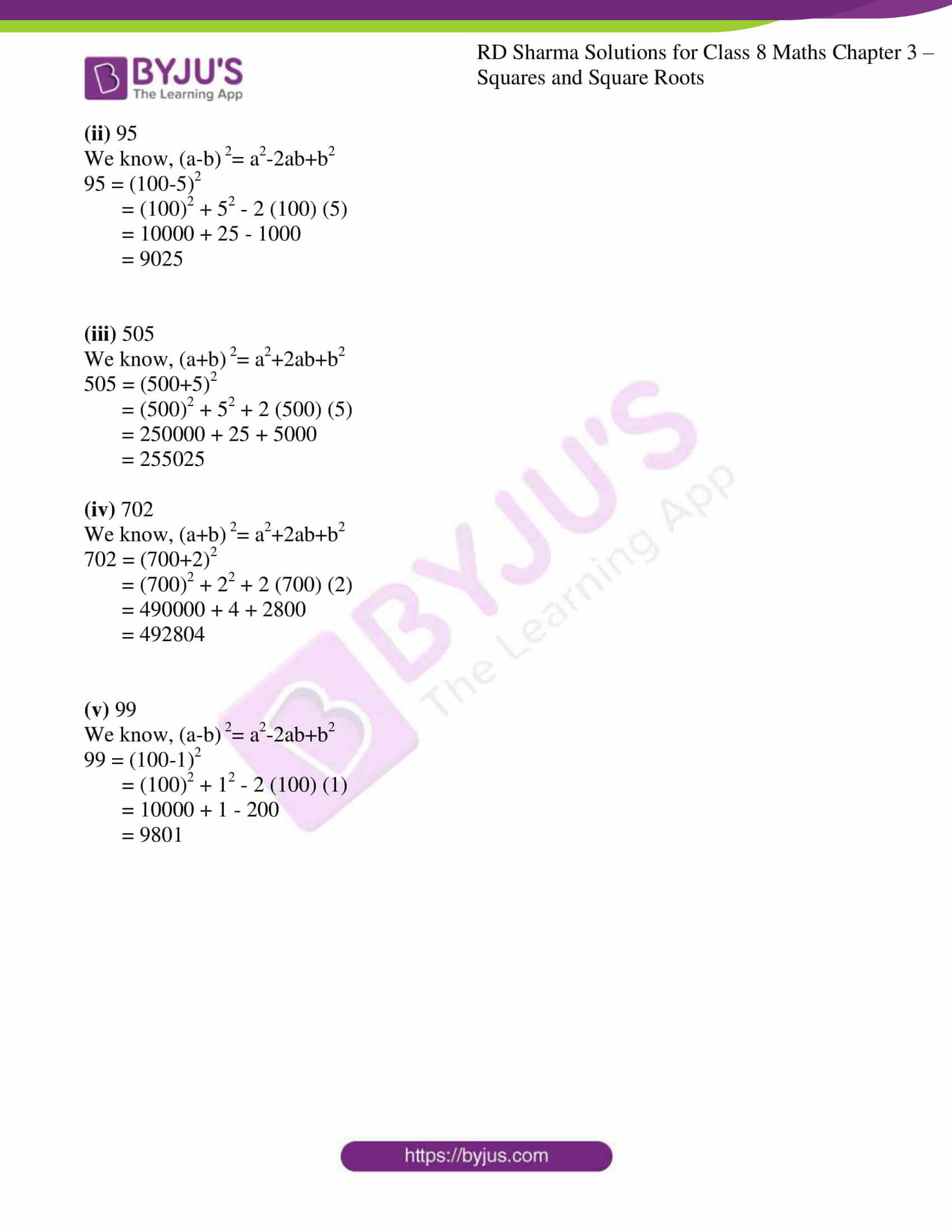### Access Answers to RD Sharma Solutions for Class 8 Maths Exercise 3.3 Chapter 3 Squares and Square Roots

#### EXERCISE 3.3 PAGE NO: 3.32

1. Find the squares of the following numbers using column method. Verify the result by finding the square using the usual multiplication:
(i) 25
(ii) 37
(iii) 54
(iv) 71
(v) 96

Solution:

(i) 25

So here, a = 2 and b = 5

 Column I Column II Column III a2 4 +2 6 2ab 20 +2 22 b2 25 6 2 5

∴ 252 = 625

Where, it can be expressed as

252 = 25× 25 = 625

(ii) 37

So here, a = 3 and b = 7

 Column I Column II Column III a2 9 +4 13 2ab 42 +4 46 b2 49 13 6 9

∴ 372 = 1369

Where, it can be expressed as

252 = 37× 37 = 1369

(iii) 54

So here, a = 5 and b = 4

 Column I Column II Column III a2 25 +4 29 2ab 40 +1 41 b2 16 29 1 6

∴ 542 = 2916

Where, it can be expressed as

542 = 54 × 54 = 2916

(iv) 71

So here, a = 7 and b = 1

 Column I Column II Column III a2 49 +1 50 2ab 14 +0 14 b2 01 50 4 1

∴ 712 = 5041

Where, it can be expressed as

712 = 71 × 71 = 5041

(v) 96

So here, a = 9 and b = 6

 Column I Column II Column III a2 81 +11 92 2ab 108 +3 111 b2 36 92 1 6

∴ 962 = 9216

Where, it can be expressed as

962 = 96 × 96 = 9216

2. Find the squares of the following numbers using diagonal method:
(i) 98
(ii) 273
(iii) 348
(iv) 295
(v) 171

Solution:

(i) 98

Step 1: Obtain the number and count the number of digits in it. Let there be n digits in the number to be squared.

Step 2: Draw square and divide it into n2 sub-squares of the same size by drawing (n – 1) horizontal and (n – 1) vertical lines.

Step 3: Draw the diagonals of each sub-square.

Step 4: Write the digits of the number to be squared along left vertical side sand top horizontal side of the squares.

Step 5: Multiply each digit on the left of the square with each digit on top of the column one-by-one. Write the units digit of the product below the diagonal and tens digit above the diagonal of the corresponding sub-square.

Step 6: Starting below the lowest diagonal sum the digits along the diagonals so obtained. Write the units digit of the sum and take carry, the tens digit (if any) to the diagonal above.

Step 7: Obtain the required square by writing the digits from the left-most side.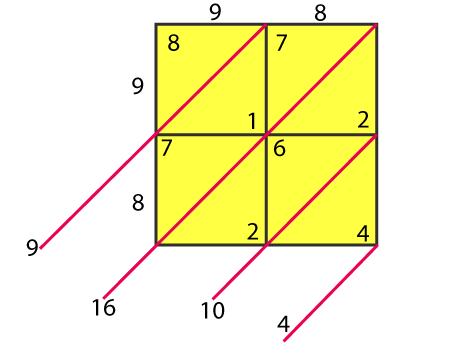∴ 982 = 9604

(ii) 273

Step 1: Obtain the number and count the number of digits in it. Let there be n digits in the number to be squared.

Step 2: Draw square and divide it into n2 sub-squares of the same size by drawing (n – 1) horizontal and (n – 1) vertical lines.

Step 3: Draw the diagonals of each sub-square.

Step 4: Write the digits of the number to be squared along left vertical side sand top horizontal side of the squares.

Step 5: Multiply each digit on the left of the square with each digit on top of the column one-by-one. Write the units digit of the product below the diagonal and tens digit above the diagonal of the corresponding sub-square.

Step 6: Starting below the lowest diagonal sum the digits along the diagonals so obtained. Write the units digit of the sum and take carry, the tens digit (if any) to the diagonal above.

Step 7: Obtain the required square by writing the digits from the left-most side.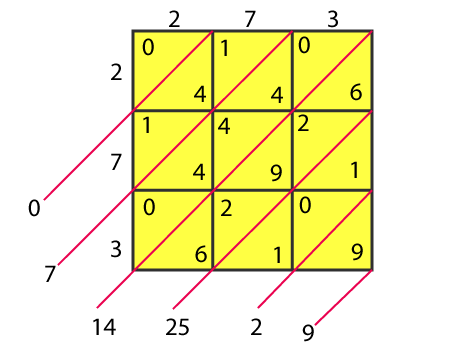∴ 2732 = 74529

(iii) 348

Step 1: Obtain the number and count the number of digits in it. Let there be n digits in the number to be squared.

Step 2: Draw square and divide it into n2 sub-squares of the same size by drawing (n – 1) horizontal and (n – 1) vertical lines.

Step 3: Draw the diagonals of each sub-square.

Step 4: Write the digits of the number to be squared along left vertical side sand top horizontal side of the squares.

Step 5: Multiply each digit on the left of the square with each digit on top of the column one-by-one. Write the units digit of the product below the diagonal and tens digit above the diagonal of the corresponding sub-square.

Step 6: Starting below the lowest diagonal sum the digits along the diagonals so obtained. Write the units digit of the sum and take carry, the tens digit (if any) to the diagonal above.

Step 7: Obtain the required square by writing the digits from the left-most side.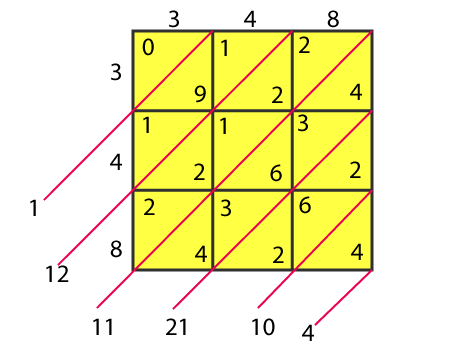∴ 3482 = 121104

(iv) 295

Step 1: Obtain the number and count the number of digits in it. Let there be n digits in the number to be squared.

Step 2: Draw square and divide it into n2 sub-squares of the same size by drawing (n – 1) horizontal and (n – 1) vertical lines.

Step 3: Draw the diagonals of each sub-square.

Step 4: Write the digits of the number to be squared along left vertical side sand top horizontal side of the squares.

Step 5: Multiply each digit on the left of the square with each digit on top of the column one-by-one. Write the units digit of the product below the diagonal and tens digit above the diagonal of the corresponding sub-square.

Step 6: Starting below the lowest diagonal sum the digits along the diagonals so obtained. Write the units digit of the sum and take carry, the tens digit (if any) to the diagonal above.

Step 7: Obtain the required square by writing the digits from the left-most side.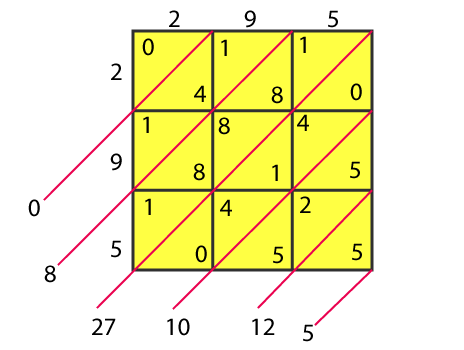∴ 2952 = 87025

(v) 171

Step 1: Obtain the number and count the number of digits in it. Let there be n digits in the number to be squared.

Step 2: Draw square and divide it into n2 sub-squares of the same size by drawing (n – 1) horizontal and (n – 1) vertical lines.

Step 3: Draw the diagonals of each sub-square.

Step 4: Write the digits of the number to be squared along left vertical side sand top horizontal side of the squares.

Step 5: Multiply each digit on the left of the square with each digit on top of the column one-by-one. Write the units digit of the product below the diagonal and tens digit above the diagonal of the corresponding sub-square.

Step 6: Starting below the lowest diagonal sum the digits along the diagonals so obtained. Write the units digit of the sum and take carry, the tens digit (if any) to the diagonal above.

Step 7: Obtain the required square by writing the digits from the left-most side.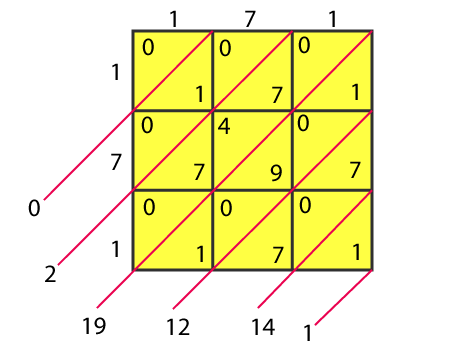∴ 1712 = 29241

3. Find the squares of the following numbers:
(i) 127

(ii) 503
(iii) 450

(iv) 862
(v) 265

Solution:

(i) 127

1272 = 127 × 127 = 16129

(ii) 503

5032 = 503 × 503 = 253009

(iii)
450

4502 = 450 × 450 = 203401

(iv) 862

8622 = 862 × 862 = 743044

(v)
265

2652 = 265 × 265 = 70225

4. Find the squares of the following numbers:
(i) 425

(ii) 575
(iii)405

(iv) 205
(v) 95

(vi) 745
(vii) 512

(viii) 995

Solution:

(i)425

4252 = 425 × 425 = 180625

(ii) 575

5752 = 575 × 575 = 330625

(iii)405

4052 = 405 × 405 = 164025

(iv) 205

2052 = 205 × 205 = 42025

(v) 95

952 = 95 × 95 = 9025

(vi) 745

7452 = 745 × 745 = 555025

(vii) 512

5122 = 512 × 512 = 262144

(viii) 995

9952 = 995 × 995 = 990025

5. Find the squares of the following numbers using the identity (a+b) 2= a2+2ab+b2:
(i) 405
(ii) 510
(iii) 1001
(iv) 209
(v) 605

Solution:

(i) 405

We know, (a+b) 2= a2+2ab+b2

405 = (400+5)2

= (400)2 + 52 + 2 (400) (5)

= 160000 + 25 + 4000

= 164025

(ii) 510

We know, (a+b) 2= a2+2ab+b2

510 = (500+10)2

= (500)2 + 102 + 2 (500) (10)

= 250000 + 100 + 10000

= 260100

(iii)
1001

We know, (a+b) 2= a2+2ab+b2

1001 = (1000+1)2

= (1000)2 + 12 + 2 (1000) (1)

= 1000000 + 1 + 2000

= 1002001

(iv)
209

We know, (a+b) 2= a2+2ab+b2

209 = (200+9)2

= (200)2 + 92 + 2 (200) (9)

= 40000 + 81 + 3600

= 43681

(v)
605

We know, (a+b) 2= a2+2ab+b2

605 = (600+5)2

= (600)2 + 52 + 2 (600) (5)

= 360000 + 25 + 6000

= 366025

6. Find the squares of the following numbers using the identity (a-b) 2= a2-2ab+b2
(i) 395

(ii) 995
(iii)495

(iv) 498
(v) 99

(vi) 999
(vii)599

Solution:

(i) 395

We know, (a-b) 2= a2-2ab+b2

395 = (400-5)2

= (400)2 + 52 – 2 (400) (5)

= 160000 + 25 – 4000

= 156025

(ii) 995

We know, (a-b) 2= a2-2ab+b2

995 = (1000-5)2

= (1000)2 + 52 – 2 (1000) (5)

= 1000000 + 25 – 10000

= 990025

(iii)
495

We know, (a-b) 2= a2-2ab+b2

495 = (500-5)2

= (500)2 + 52 – 2 (500) (5)

= 250000 + 25 – 5000

= 245025

(iv) 498

We know, (a-b) 2= a2-2ab+b2

498 = (500-2)2

= (500)2 + 22 – 2 (500) (2)

= 250000 + 4 – 2000

= 248004

(v)
99

We know, (a-b) 2= a2-2ab+b2

99 = (100-1)2

= (100)2 + 12 – 2 (100) (1)

= 10000 + 1 – 200

= 9801

(vi) 999

We know, (a-b) 2= a2-2ab+b2

999 = (1000-1)2

= (1000)2 + 12 – 2 (1000) (1)

= 1000000 + 1 – 2000

= 998001

(vii)
599

We know, (a-b) 2= a2-2ab+b2

599 = (600-1)2

= (600)2 + 12 – 2 (600) (1)

= 360000 + 1 – 1200

= 358801

7. Find the squares of the following numbers by visual method:
(i) 52

(ii) 95
(iii) 505

(iv) 702
(v) 99

Solution:

(i) 52

We know, (a+b) 2= a2+2ab+b2

52 = (50+2)2

= (50)2 + 22 + 2 (50) (2)

= 2500 + 4 + 200

= 2704

(ii) 95

We know, (a-b) 2= a2-2ab+b2

95 = (100-5)2

= (100)2 + 52 – 2 (100) (5)

= 10000 + 25 – 1000

= 9025

(iii)
505

We know, (a+b) 2= a2+2ab+b2

505 = (500+5)2

= (500)2 + 52 + 2 (500) (5)

= 250000 + 25 + 5000

= 255025

(iv) 702

We know, (a+b) 2= a2+2ab+b2

702 = (700+2)2

= (700)2 + 22 + 2 (700) (2)

= 490000 + 4 + 2800

= 492804

(v)
99

We know, (a-b) 2= a2-2ab+b2

99 = (100-1)2

= (100)2 + 12 – 2 (100) (1)

= 10000 + 1 – 200

= 9801

## RD Sharma Solutions for Class 8 Maths Exercise 3.3 Chapter 3 – Squares and Square Roots

Exercise 3.3 of RD Sharma Solutions for Chapter 3 Squares and Square Roots, this exercise mainly deals with the basic concepts related to methods for squaring a two-digit number. Here are a few methods,

### Column method for squaring a two-digit number

• Visual method for squaring a two-digit number
• Diagonal method for squaring a two-digit number

The RD Sharma Solutions can help students in practising and learning each and every concept as it provides solutions to all questions asked in RD Sharma textbook.